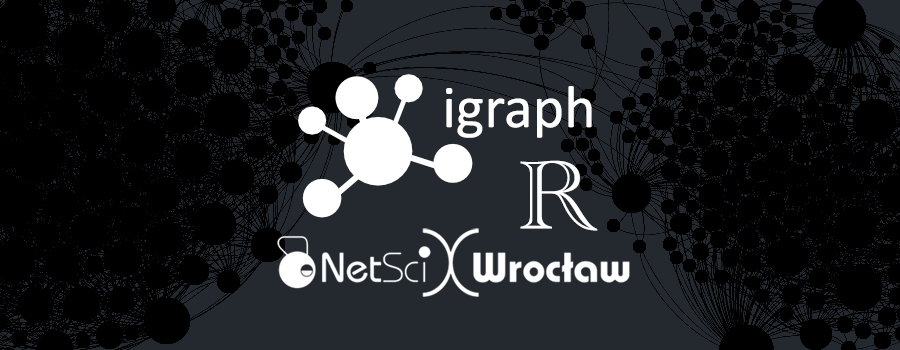This tutorial covers basics of network analysis and visualization with the R package igraph (maintained by Gabor Csardi and Tamas Nepusz). The igraph library provides versatile options for descriptive network analysis and visualization in R, Python, and C/C++. This workshop will focus on the R implementation. You will need an R installation, and RStudio. You should also install the latest version of `igraph` for R:

`` install.packages("igraph")``

# 1. A quick reminder of R basics

Before we start working with networks, we will go through a quick introduction/reminder of some simple tasks and principles in R.

## 1.1 Assignment

You can assign a value to an object using `assign()`, `<-`, or `=`.

``````x <- 3         # Assignment

x              # Evaluate the expression and print result

y <- 4         # Assignment

y + 5          # Evaluation, y remains 4

z <- x + 17*y  # Assignment

z              # Evaluation``````
``````rm(z)          # Remove z: deletes the object.

z              # Error!``````

## 1.2 Value comparisons

We can use the standard operators `<`, `>`, `<=`, `>=`, `==`(equality) and `!=` (inequality). Comparisons return Boolean values: `TRUE` or `FALSE` (often abbreviated to just `T` and `F`).

``````2==2  # Equality

2!=2  # Inequality

x <= y # less than or equal: "<", ">", and ">=" also work``````

## 1.3 Special constants

Special constants include:

• NA for missing or undefined data
• NULL for empty object (e.g. null/empty lists)
• Inf and -Inf for positive and negative infinity
• NaN for results that cannot be reasonably defined
``````# NA - missing or undefined data

5 + NA      # When used in an expression, the result is generally NA

is.na(5+NA) # Check if missing

# NULL - an empty object, e.g. a null/empty list

10 + NULL     # use returns an empty object (length zero)

is.null(NULL) # check if NULL``````

Inf and -Inf represent positive and negative infinity. They can be returned by mathematical operations like division of a number by zero:

``````5/0

is.finite(5/0) # Check if a number is finite (it is not).``````

NaN (Not a Number) - the result of an operation that cannot be reasonably defined, such as dividing zero by zero.

``````0/0

is.nan(0/0)``````

## 1.4 Vectors

Vectors can be constructed by combining their elements with the important R function `c()`.

``````v1 <- c(1, 5, 11, 33)       # Numeric vector, length 4

v2 <- c("hello","world")    # Character vector, length 2 (a vector of strings)

v3 <- c(TRUE, TRUE, FALSE)  # Logical vector, same as c(T, T, F)``````

Combining different types of elements in one vector will coerce the elements to the least restrictive type:

``v4 <- c(v1,v2,v3,"boo")     # All elements turn into strings``

Other ways to create vectors include:

``````v <- 1:7         # same as c(1,2,3,4,5,6,7)

v <- rep(0, 77)  # repeat zero 77 times: v is a vector of 77 zeroes

v <- rep(1:3, times=2) # Repeat 1,2,3 twice

v <- rep(1:10, each=2) # Repeat each element twice

v <- seq(10,20,2) # sequence: numbers between 10 and 20, in jumps of 2

v1 <- 1:5         # 1,2,3,4,5

v2 <- rep(1,5)    # 1,1,1,1,1 ``````

Check the length of a vector:

``````length(v1)

length(v2)``````

Element-wise operations:

``````v1 + v2      # Element-wise addition

v1 + 1       # Add 1 to each element

v1 * 2       # Multiply each element by 2

v1 + c(1,7)  # This doesn't work: (1,7) is a vector of different length``````

Mathematical operations:

``````sum(v1)      # The sum of all elements

mean(v1)     # The average of all elements

sd(v1)       # The standard deviation

cor(v1,v1*5) # Correlation between v1 and v1*5 ``````

Logical operations:

``````v1 > 2       # Each element is compared to 2, returns logical vector

v1==v2       # Are corresponding elements equivalent, returns logical vector.

v1!=v2       # Are corresponding elements *not* equivalent? Same as !(v1==v2)

(v1>2) | (v2>0)   # | is the boolean OR, returns a vector.

(v1>2) & (v2>0)   # & is the boolean AND, returns a vector.

(v1>2) || (v2>0)  # || is the boolean OR, returns a single value

(v1>2) && (v2>0)  # && is the boolean AND, ditto``````

Vector elements:

``````v1             # third element of v1

v1[2:4]           # elements 2, 3, 4 of v1

v1[c(1,3)]        # elements 1 and 3 - note that your indexes are a vector

v1[c(T,T,F,F,F)]  # elements 1 and 2 - only the ones that are TRUE

v1[v1>3]          # v1>3 is a logical vector TRUE for elements >3``````

Note that the indexing in R starts from `1`, a fact known to confuse and upset people used to languages that index from `0`.

To add more elements to a vector, simply assign them values.

``v1[6:10] <- 6:10``

We can also directly assign the vector a length:

``length(v1) <- 15 # the last 5 elements are added as missing data: NA``

## 1.5 Factors

Factors are used to store categorical data.

``````eye.col.v <- c("brown", "green", "brown", "blue", "blue", "blue")         #vector

eye.col.f <- factor(c("brown", "green", "brown", "blue", "blue", "blue")) #factor

eye.col.v``````
``##  "brown" "green" "brown" "blue"  "blue"  "blue"``
``eye.col.f``
``````##  brown green brown blue  blue  blue

## Levels: blue brown green``````

R will identify the different levels of the factor - e.g. all distinct values. The data is stored internally as integers - each number corresponding to a factor level.

``levels(eye.col.f)  # The levels (distinct values) of the factor (categorical var)``
``##  "blue"  "brown" "green"``
``as.numeric(eye.col.f)  # As numeric values: 1 is  blue, 2 is brown, 3 is green``
``##  2 3 2 1 1 1``
``as.numeric(eye.col.v)  # The character vector can not be coerced to numeric``
``## Warning: NAs introduced by coercion``
``##  NA NA NA NA NA NA``
``as.character(eye.col.f)  ``
``##  "brown" "green" "brown" "blue"  "blue"  "blue"``
``as.character(eye.col.v) ``
``##  "brown" "green" "brown" "blue"  "blue"  "blue"``

## 1.6 Matrces & Arrays

A matrix is a vector with dimensions:

``````m <- rep(1, 20)   # A vector of 20 elements, all 1

dim(m) <- c(5,4)  # Dimensions set to 5 & 4, so m is now a 5x4 matrix``````

Creating a matrix using `matrix():`

``````m <- matrix(data=1, nrow=5, ncol=4)  # same matrix as above, 5x4, full of 1s

m <- matrix(1,5,4)                       # same matrix as above

dim(m)                               # What are the dimensions of m?``````
``##  5 4``

Creating a matrix by combining vectors:

``````m <- cbind(1:5, 5:1, 5:9)  # Bind 3 vectors as columns, 5x3 matrix

m <- rbind(1:5, 5:1, 5:9)  # Bind 3 vectors as rows, 3x5 matrix``````

Selecting matrix elements:

``````m <- matrix(1:10,10,10)

m[2,3]  # Matrix m, row 2, column 3 - a single cell

m[2,]   # The whole second row of m as a vector

m[,2]   # The whole second column of m as a vector

m[1:2,4:6] # submatrix: rows 1 and 2, columns 4, 5 and 6

m[-1,]     # all rows *except* the first one``````

Other operations with matrices:

``````# Are elements in row 1 equivalent to corresponding elements from column 1:

m[1,]==m[,1]

# A logical matrix: TRUE for m elements >3, FALSE otherwise:

m>3

# Selects only TRUE elements - that is ones greater than 3:

m[m>3]``````
``````t(m)          # Transpose m

m <- t(m)     # Assign m the transposed m

m %*% t(m)    # %*% does matrix multiplication

m * m         # * does element-wise multiplication``````

Arrays are used when we have more than 2 dimensions. We can create them using the `array()` function:

``````a <- array(data=1:18,dim=c(3,3,2)) # 3d with dimensions 3x3x2

a <- array(1:18,c(3,3,2))          # the same array``````

## 1.7 Lists

Lists are collections of objects. A single list can contain all kinds of elements - character strings, numeric vectors, matrices, other lists, and so on. The elements of lists are often named for easier access.

``````l1 <- list(boo=v1,foo=v2,moo=v3,zoo="Animals!")  # A list with four components

l2 <- list(v1,v2,v3,"Animals!")``````

Create an empty list:

``````l3 <- list()

l4 <- NULL``````

Accessing list elements:

``````l1["boo"]   # Access boo with single brackets: this returns a list.

l1[["boo"]] # Access boo with double brackets: this returns the numeric vector

l1[]     # Returns the first component of the list, equivalent to above.

l1\$boo      # Named elements can be accessed with the \$ operator, as with [[]]``````

Adding more elements to a list:

``````l3[] <- 11 # add an element to the empty list l3

l4[] <- c(22, 23) # add a vector as element 3 in the empty list l4. ``````

Since we added element 3 to the list `l4`above, elements 1 and 2 will be generated and empty (NULL).

``````l1[] <- "More elements!" # The list l1 had 4 elements, we're adding a 5th here.

l1[] <- 1:11 ``````

We added an 8th element, but not 6th and 7th to the list`l1` above. Elements number 6 and 7 will be created empty (NULL).

``l1\$Something <- "A thing"  # Adds a ninth element - "A thing", named "Something"``

## 1.8 Data Frames

The data frame is a special kind of list used for storing dataset tables. Think of rows as cases, columns as variables. Each column is a vector or factor.

Creating a dataframe:

``````dfr1 <- data.frame( ID=1:4,

FirstName=c("John","Jim","Jane","Jill"),

Female=c(F,F,T,T),

Age=c(22,33,44,55) )

dfr1\$FirstName   # Access the second column of dfr1. ``````
``````##  John Jim  Jane Jill

## Levels: Jane Jill Jim John``````

Notice that R thinks that `dfr1\$FirstName` is a categorical variable and so it’s treating it like a factor, not a character vector. Let’s get rid of the factor by telling R to treat ‘FirstName’ as a vector:

``dfr1\$FirstName <- as.vector(dfr1\$FirstName)``

Alternatively, you can tell R you don’t like factors from the start using `stringsAsFactors=FALSE`

``````dfr2 <- data.frame(FirstName=c("John","Jim","Jane","Jill"), stringsAsFactors=F)

dfr2\$FirstName   # Success: not a factor.``````
``##  "John" "Jim"  "Jane" "Jill"``

Access elements of the data frame:

``````dfr1[1,]   # First row, all columns

dfr1[,1]   # First column, all rows

dfr1\$Age   # Age column, all rows

dfr1[1:2,3:4] # Rows 1 and 2, columns 3 and 4 - the gender and age of John & Jim

dfr1[c(1,3),] # Rows 1 and 3, all columns``````

Find the names of everyone over the age of 30 in the data:

``dfr1[dfr1\$Age>30,2]``
``##  "Jim"  "Jane" "Jill"``

Find the average age of all females in the data:

``mean ( dfr1[dfr1\$Female==TRUE,4] )``
``##  49.5``

## 1.9 Flow Control and loops

The controls and loops in R are fairly straightforward (see below). They determine if a block of code will be executed, and how many times. Blocks of code in R are enclosed in curly brackets `{}`.

``````# if (condition) expr1 else expr2

x <- 5; y <- 10

if (x==0) y <- 0 else y <- y/x #

y``````
``##  2``
``````# for (variable in sequence) expr

ASum <- 0; AProd <- 1

for (i in 1:x)

{

ASum <- ASum + i

AProd <- AProd * i

}

ASum  # equivalent to sum(1:x)``````
``##  15``
``AProd # equivalemt to prod(1:x)``
``##  120``
``````# while (condintion) expr

while (x > 0) {print(x); x <- x-1;}

# repeat expr, use break to exit the loop

repeat { print(x); x <- x+1; if (x>10) break}``````

## 1.10 R plots and colors

In most R functions, you can use named colors, hex, or RGB values. In the simple base R plot chart below, `x` and `y` are the point coordinates, `pch` is the point symbol shape, `cex` is the point size, and `col` is the color. To see the parameters for plotting in base R, check out `?par`

``````plot(x=1:10, y=rep(5,10), pch=19, cex=3, col="dark red")

points(x=1:10, y=rep(6, 10), pch=19, cex=3, col="557799")

points(x=1:10, y=rep(4, 10), pch=19, cex=3, col=rgb(.25, .5, .3))``````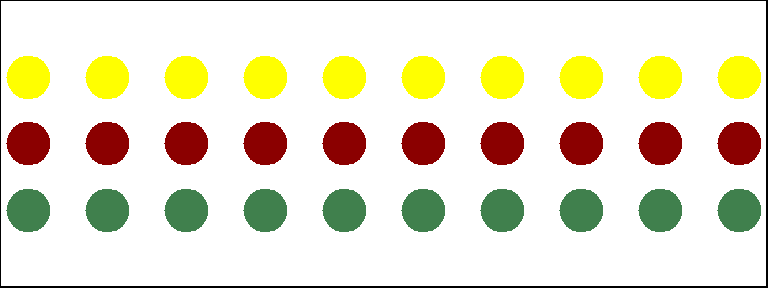You may notice that RGB here ranges from 0 to 1. While this is the R default, you can also set it for to the 0-255 range using something like `rgb(10, 100, 100, maxColorValue=255)`.

We can set the opacity/transparency of an element using the parameter `alpha` (range 0-1):

``plot(x=1:5, y=rep(5,5), pch=19, cex=12, col=rgb(.25, .5, .3, alpha=.5), xlim=c(0,6))  ``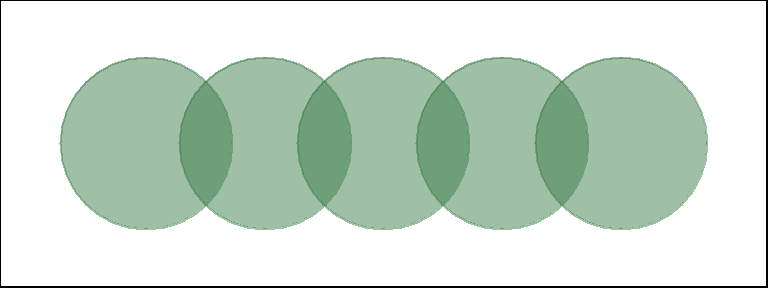If we have a hex color representation, we can set the transparency alpha using `adjustcolor` from package `grDevices`. For fun, let’s also set the plot background to gray using the `par()` function for graphical parameters.

``````par(bg="gray40")

plot(x=1:5, y=rep(5,5), pch=19, cex=12, col=col.tr, xlim=c(0,6)) ``````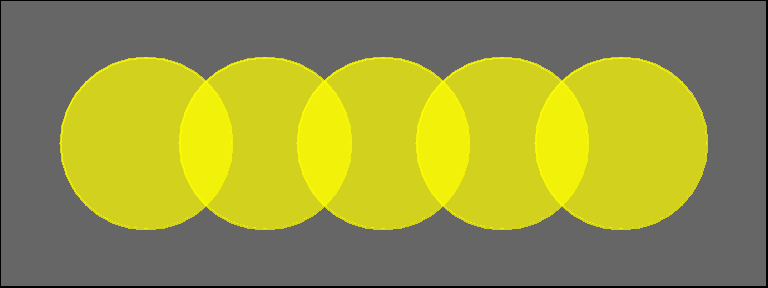If you plan on using the built-in color names, here’s how to list all of them:

``````colors()                          # List all named colors

grep("blue", colors(), value=T)   # Colors that have "blue" in the name``````

In many cases, we need a number of contrasting colors, or multiple shades of a color. R comes with some predefined palette function that can generate those for us. For example:

``````pal1 <- heat.colors(5, alpha=1)   #  5 colors from the heat palette, opaque

pal2 <- rainbow(5, alpha=.5)      #  5 colors from the heat palette, transparent

plot(x=1:10, y=1:10, pch=19, cex=5, col=pal1)``````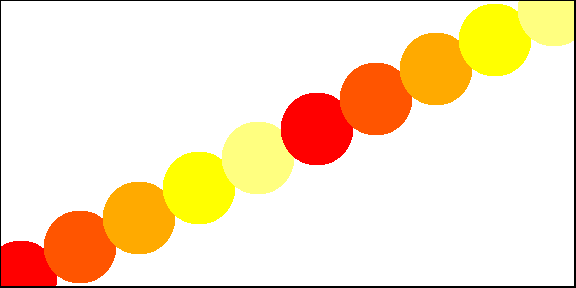``plot(x=1:10, y=1:10, pch=19, cex=5, col=pal2)``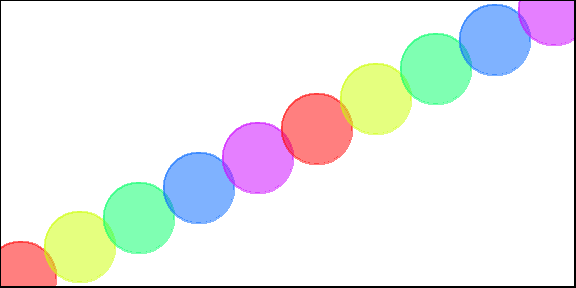We can also generate our own gradients using `colorRampPalette`. Note that `colorRampPalette` returns a function that we can use to generate as many colors from that palette as we need.

``````palf <- colorRampPalette(c("gray80", "dark red"))

plot(x=10:1, y=1:10, pch=19, cex=5, col=palf(10)) ``````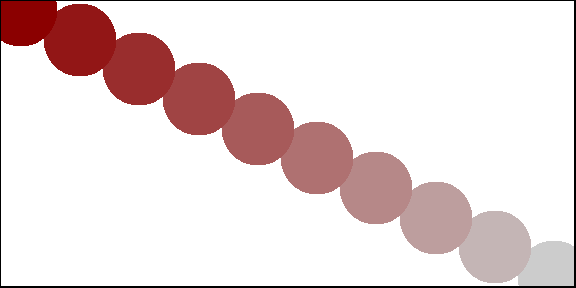To add transparency to colorRampPalette, you need to use a parameter `alpha=TRUE`:

``````palf <- colorRampPalette(c(rgb(1,1,1, .2),rgb(.8,0,0, .7)), alpha=TRUE)

plot(x=10:1, y=1:10, pch=19, cex=5, col=palf(10)) ``````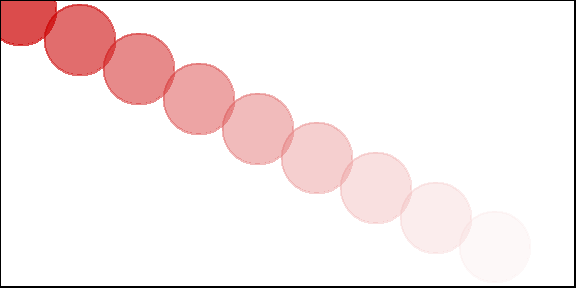## 1.11 R troubleshooting

While I generate many (and often very creative) errors in R, there are three simple things that will most often go wrong for me. Those include:

1. Capitalization. R is case sensitive - a graph vertex named “Jack” is not the same as one named “jack”. The function `rowSums` won’t work if spelled as `rowsums` or `RowSums`.

2. Object class. While many functions are willing to take anything you throw at them, some will still surprisingly require character vector or a factor instead of a numeric vector, or a matrix instead of a data frame. Functions will also occasionally return results in an unexpected formats.

3. Package namespaces. Occasionally problems will arise when different packages contain functions with the same name. R may warn you about this by saying something like “The following object(s) are masked from ‘package:igraph’ as you load a package. One way to deal with this is to call functions from a package explicitly using `::`. For instance, if function `blah()` is present in packages A and B, you can call `A::blah` and `B::blah`. In other cases the problem is more complicated, and you may have to load packages in certain order, or not use them together at all. For example (and pertinent to this workshop), `igraph` and `Statnet` packages cause some problems when loaded at the same time. It is best to detach one before loading the other.

`````` library(igraph)          # load a package

detach(package:igraph)   # detach a package``````

For more advanced troubleshooting, check out `try()`, `tryCatch()`, and `debug()`.

# 2. Networks in igraph

``````rm(list = ls()) # Remove all the objects we created so far.

library(igraph) # Load the igraph package``````

## 2.1 Create networks

The code below generates an undirected graph with three edges. The numbers are interpreted as vertex IDs, so the edges are 1–>2, 2–>3, 3–>1.

``````g1 <- graph( edges=c(1,2, 2,3, 3, 1), n=3, directed=F )

plot(g1) # A simple plot of the network - we'll talk more about plots later``````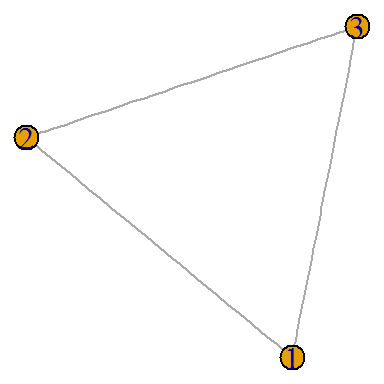``class(g1)``
``##  "igraph"``
``g1``
``````## IGRAPH U--- 3 3 --

## + edges:

##  1--2 2--3 1--3``````
``````# Now with 10 vertices, and directed by default:

g2 <- graph( edges=c(1,2, 2,3, 3, 1), n=10 )

plot(g2)   ``````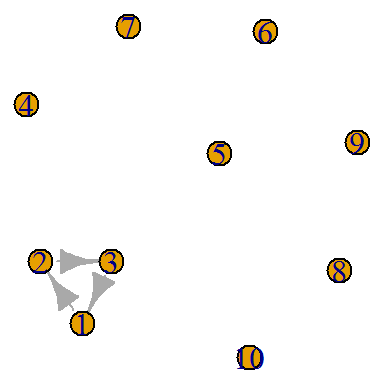``g2``
``````## IGRAPH D--- 10 3 --

## + edges:

##  1->2 2->3 3->1``````
``````g3 <- graph( c("John", "Jim", "Jim", "Jill", "Jill", "John")) # named vertices

# When the edge list has vertex names, the number of nodes is not needed

plot(g3)``````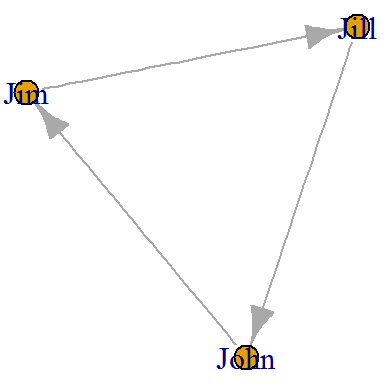``g3``
``````## IGRAPH DN-- 3 3 --

## + attr: name (v/c)

## + edges (vertex names):

##  John->Jim  Jim ->Jill Jill->John``````
``````g4 <- graph( c("John", "Jim", "Jim", "Jack", "Jim", "Jack", "John", "John"),

isolates=c("Jesse", "Janis", "Jennifer", "Justin") )

# In named graphs we can specify isolates by providing a list of their names.

plot(g4, edge.arrow.size=.5, vertex.color="gold", vertex.size=15,

vertex.frame.color="gray", vertex.label.color="black",

vertex.label.cex=0.8, vertex.label.dist=2, edge.curved=0.2) ``````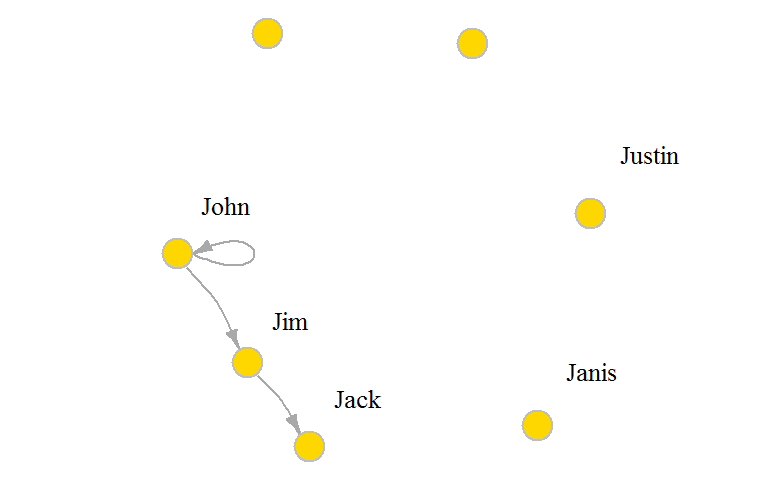Small graphs can also be generated with a description of this kind: `-` for undirected tie, `+-` or `-+` for directed ties pointing left & right, `++` for a symmetric tie, and “:” for sets of vertices.

``plot(graph_from_literal(a---b, b---c)) # the number of dashes doesn't matter``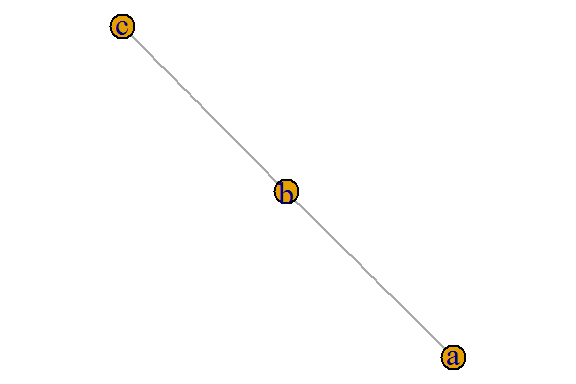``plot(graph_from_literal(a--+b, b+--c))``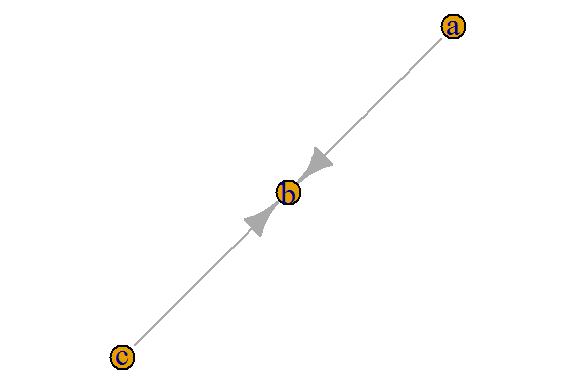``plot(graph_from_literal(a+-+b, b+-+c)) ``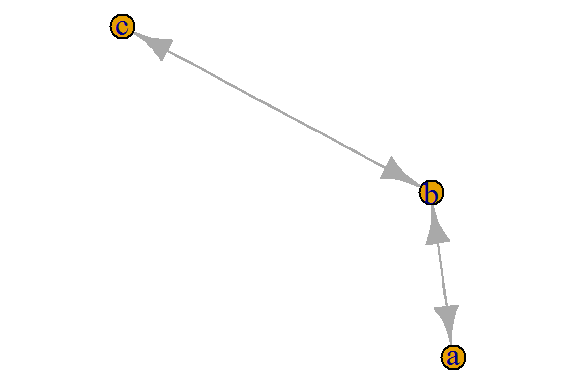``plot(graph_from_literal(a:b:c---c:d:e))``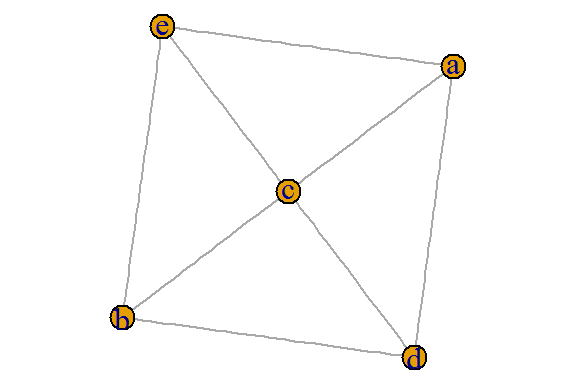``````gl <- graph_from_literal(a-b-c-d-e-f, a-g-h-b, h-e:f:i, j)

plot(gl)``````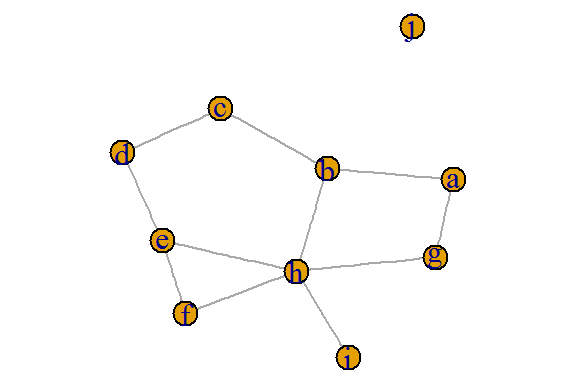## 2.2 Edge, vertex, and network attributes

Access vertices and edges:

``E(g4) # The edges of the object``
``````## + 4/4 edges (vertex names):

##  John->Jim  Jim ->Jack Jim ->Jack John->John``````
``V(g4) # The vertices of the object``
``````## + 7/7 vertices, named:

##  John     Jim      Jack     Jesse    Janis    Jennifer Justin``````

You can also examine the network matrix directly:

``g4[]``
``````## 7 x 7 sparse Matrix of class "dgCMatrix"

##          John Jim Jack Jesse Janis Jennifer Justin

## John        1   1    .     .     .        .      .

## Jim         .   .    2     .     .        .      .

## Jack        .   .    .     .     .        .      .

## Jesse       .   .    .     .     .        .      .

## Janis       .   .    .     .     .        .      .

## Jennifer    .   .    .     .     .        .      .

## Justin      .   .    .     .     .        .      .``````
``g4[1,] ``
``````##     John      Jim     Jack    Jesse    Janis Jennifer   Justin

##        1        1        0        0        0        0        0``````

Add attributes to the network, vertices, or edges:

``V(g4)\$name # automatically generated when we created the network.``
``````##  "John"     "Jim"      "Jack"     "Jesse"    "Janis"    "Jennifer"

##  "Justin"``````
``````V(g4)\$gender <- c("male", "male", "male", "male", "female", "female", "male")

E(g4)\$type <- "email" # Edge attribute, assign "email" to all edges

E(g4)\$weight <- 10    # Edge weight, setting all existing edges to 10``````

Examine attributes:

``edge_attr(g4)``
``````## \$type

##  "email" "email" "email" "email"

##

## \$weight

##  10 10 10 10``````
``vertex_attr(g4)``
``````## \$name

##  "John"     "Jim"      "Jack"     "Jesse"    "Janis"    "Jennifer"

##  "Justin"

##

## \$gender

##  "male"   "male"   "male"   "male"   "female" "female" "male"``````
``graph_attr(g4)``
``## named list()``

Another way to set attributes (you can similarly use `set_edge_attr()`, `set_vertex_attr()`, etc.):

``````g4 <- set_graph_attr(g4, "name", "Email Network")

g4 <- set_graph_attr(g4, "something", "A thing")

graph_attr_names(g4)``````
``##  "name"      "something"``
``graph_attr(g4, "name")``
``##  "Email Network"``
``graph_attr(g4)``
``````## \$name

##  "Email Network"

##

## \$something

##  "A thing"``````
``````g4 <- delete_graph_attr(g4, "something")

graph_attr(g4)``````
``````## \$name

##  "Email Network"``````
``````plot(g4, edge.arrow.size=.5, vertex.label.color="black", vertex.label.dist=1.5,

vertex.color=c( "pink", "skyblue")[1+(V(g4)\$gender=="male")] ) ``````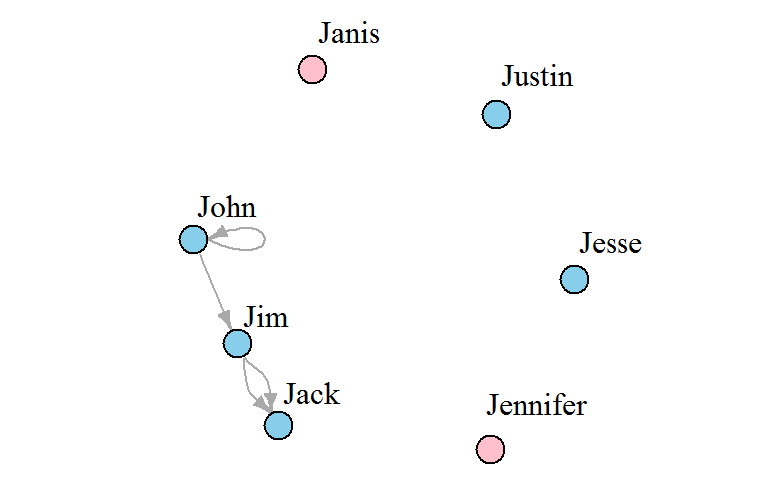The graph `g4` has two edges going from Jim to Jack, and a loop from John to himself. We can simplify our graph to remove loops & multiple edges between the same nodes. Use `edge.attr.comb` to indicate how edge attributes are to be combined - possible options include `sum`, `mean`, `prod` (product), `min`, `max`, `first`/`last` (selects the first/last edge’s attribute). Option “ignore” says the attribute should be disregarded and dropped.

``````g4s <- simplify( g4, remove.multiple = T, remove.loops = F,

edge.attr.comb=c(weight="sum", type="ignore") )

plot(g4s, vertex.label.dist=1.5)``````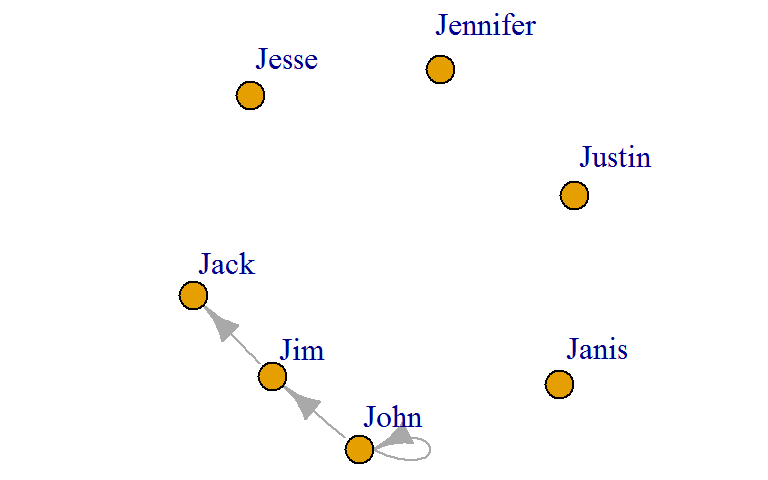``g4s``
``````## IGRAPH DNW- 7 3 -- Email Network

## + attr: name (g/c), name (v/c), gender (v/c), weight (e/n)

## + edges (vertex names):

##  John->John John->Jim  Jim ->Jack``````

The description of an igraph object starts with up to four letters:

1. D or U, for a directed or undirected graph
2. N for a named graph (where nodes have a `name` attribute)
3. W for a weighted graph (where edges have a `weight` attribute)
4. B for a bipartite (two-mode) graph (where nodes have a `type` attribute)

The two numbers that follow (7 5) refer to the number of nodes and edges in the graph. The description also lists node & edge attributes, for example:

• `(g/c)` - graph-level character attribute
• `(v/c)` - vertex-level character attribute
• `(e/n)` - edge-level numeric attribute

## 2.3 Specific graphs and graph models

Empty graph

``````eg <- make_empty_graph(40)

plot(eg, vertex.size=10, vertex.label=NA)``````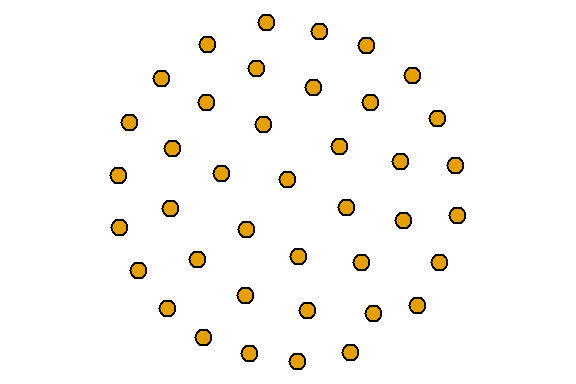Full graph

``````fg <- make_full_graph(40)

plot(fg, vertex.size=10, vertex.label=NA)``````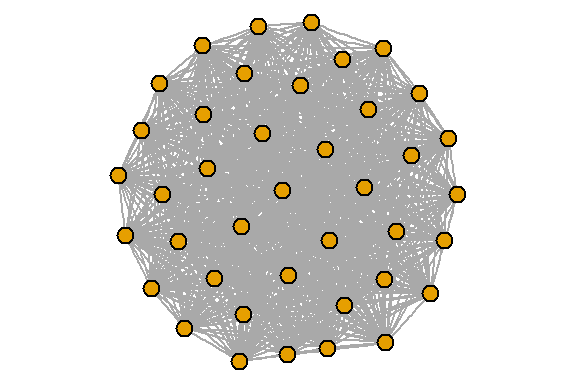Simple star graph

``````st <- make_star(40)

plot(st, vertex.size=10, vertex.label=NA) ``````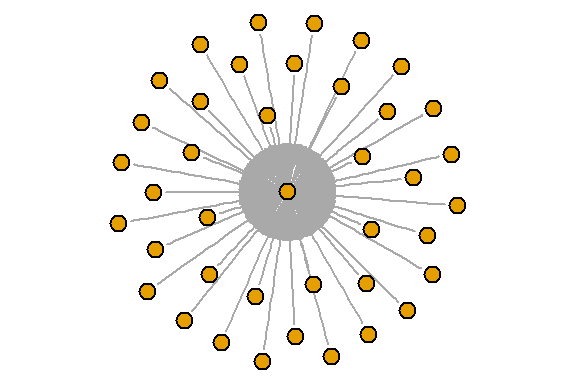Tree graph

``````tr <- make_tree(40, children = 3, mode = "undirected")

plot(tr, vertex.size=10, vertex.label=NA) ``````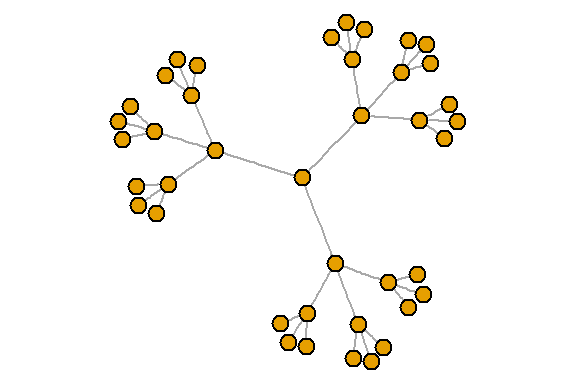Ring graph

``````rn <- make_ring(40)

plot(rn, vertex.size=10, vertex.label=NA)``````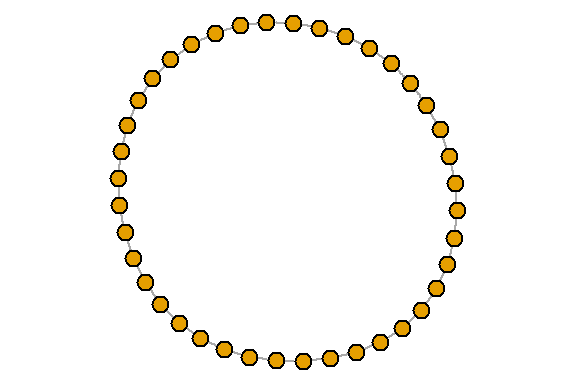Erdos-Renyi random graph model
(‘n’ is number of nodes, ‘m’ is the number of edges).

``````er <- sample_gnm(n=100, m=40)

plot(er, vertex.size=6, vertex.label=NA)  ``````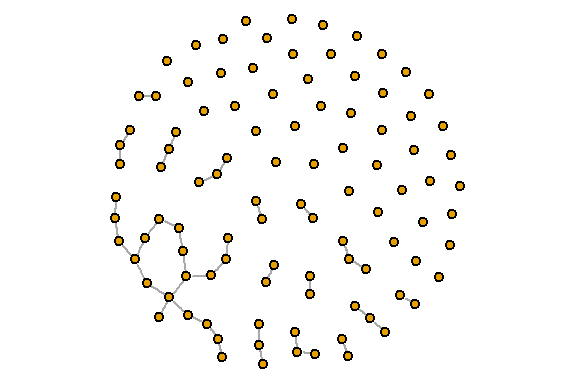Watts-Strogatz small-world model
Creates a lattice (with `dim` dimensions and `size` nodes across dimension) and rewires edges randomly with probability `p`. The neighborhood in which edges are connected is `nei`. You can allow `loops` and `multiple` edges.

``````sw <- sample_smallworld(dim=2, size=10, nei=1, p=0.1)

plot(sw, vertex.size=6, vertex.label=NA, layout=layout_in_circle)``````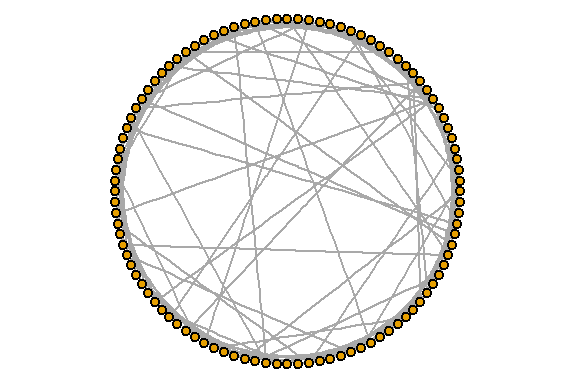Barabasi-Albert preferential attachment model for scale-free graphs
(`n` is number of nodes,